### IMO Shortlist 1996 problem G2

Kvaliteta:
Avg: 4,0
Težina:
Avg: 6,0
Let$P$ be a point inside a triangle$ABC$ such that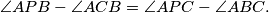Let$D$,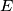$E$ be the incenters of triangles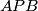$APB$,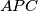$APC$, respectively. Show that the lines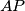$AP$,$BD$,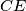$CE$ meet at a point.
Izvor: Međunarodna matematička olimpijada, shortlist 1996